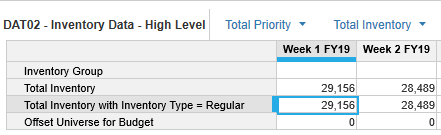# Sum based on specific value from another column?Hi All,

For line 3 in my module, I am trying to sum ONLY the lines where Total Inventory = Regular (Dimension), how should I setup this equation?Tagged:

•If you don't already have a SYS module for the Inventory dimension, create one.  Create a line item (formatted as a boolean) and name it "Sum for Regular".  Click the boolean to true for all members that you want to be in this calculation (could be one, could be many).

In your module, the formula would be: If SYS Inventory.Sum for Regular then do the sum else 0.

Hope this helps,

Rob